# Cross-tab reports

The cross-tabulation tool allows you to measure the interaction between two questions (variables). The table will only show respondents that answered both of the questions, meaning the frequencies shown may differ from a standard frequency table. The cross-tab report will also show Pearson’s Chi-Square Statistics, which shows the level of correlation between the variables using the chi-square, p-value, and degrees of freedom.

• My Surveys (Select Survey) » Analytics
• Click on Cross-Tabulation under Analysis
•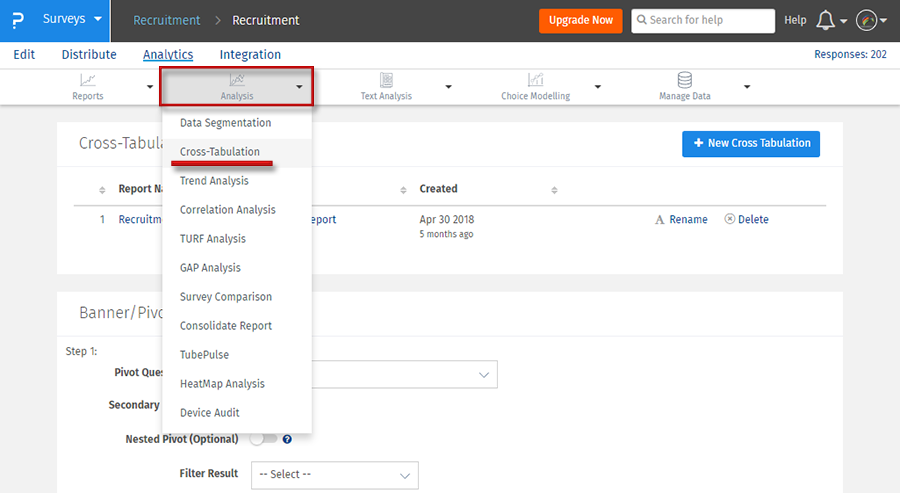• Select a row question and a column question from the respective dropdowns.
• The cross-tab table will be generated, along with Pearson’s Chi-Square Statistics and a Significant Cells for both questions.
•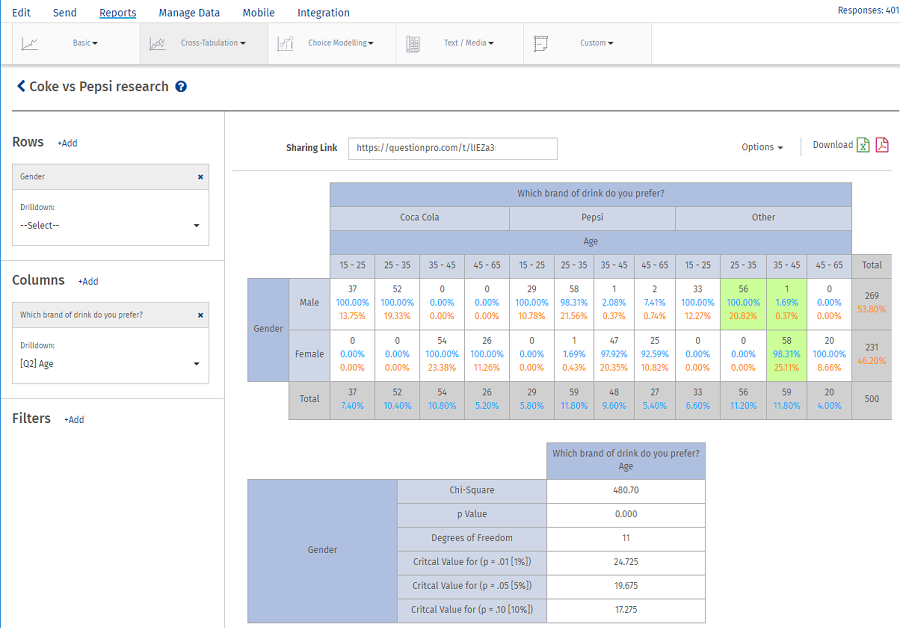## Can I filter the data that will be used to generate the report?

Yes, you can filter the data based on the following:

1. Responses to Questions
2. System Variables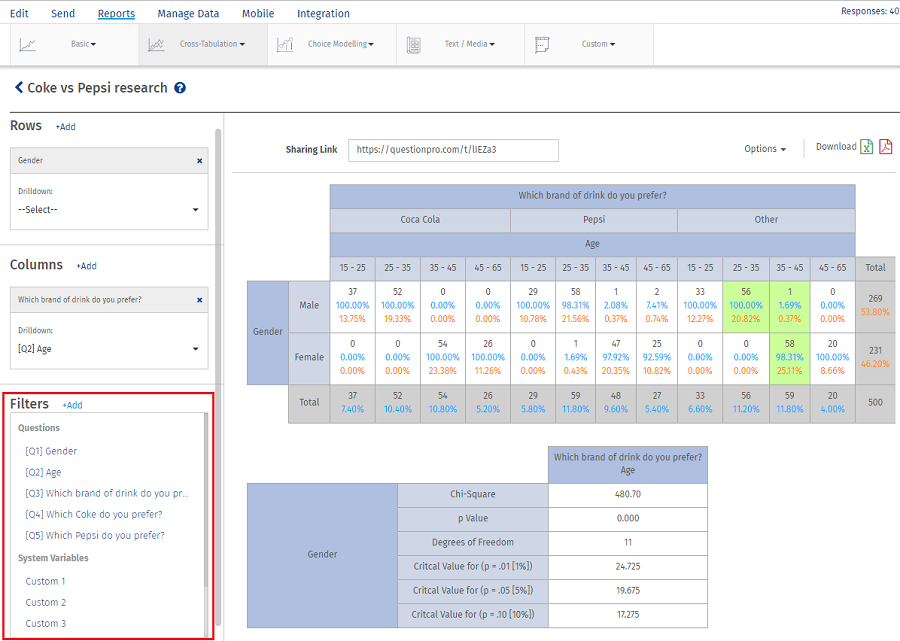## Can I share the report?

Yes, you can share the report using the sharing link.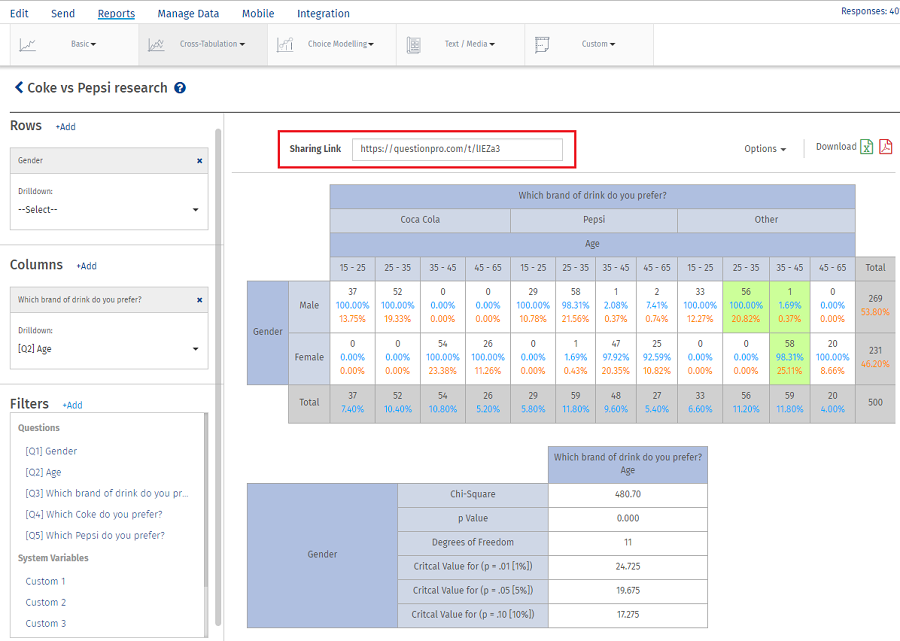1. PDF
2. Excel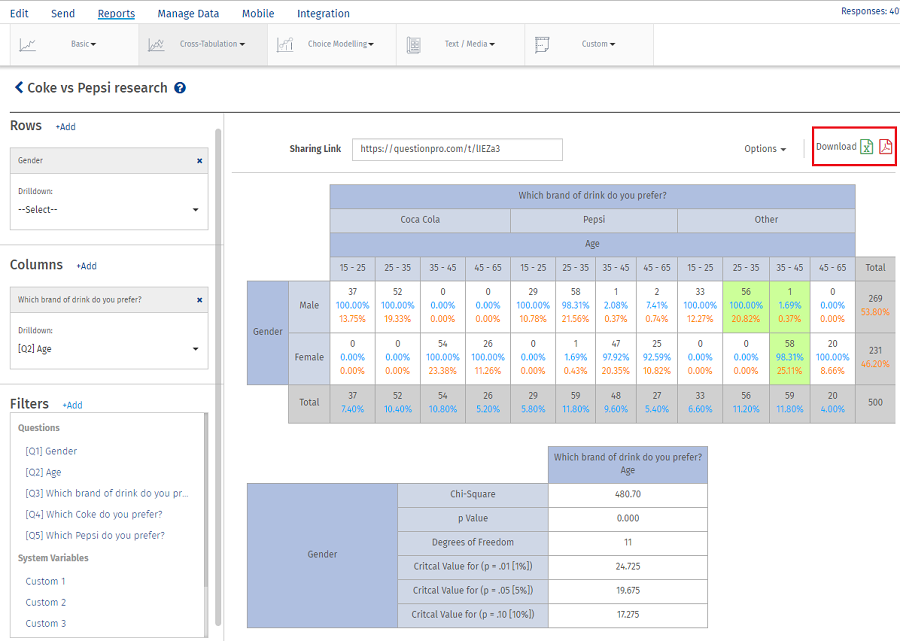## What are row and column percentages?

• Row Percents: The percent of the row total that each cell represents.

• Row Percentage = (Cell frequency/Row Total)*100
= 37/269
=13.75%

• Column Percents: The percent of the column total that each cell represents.

• Column Percentage = (Cell frequency/Column Total)*100
= 37/37
= 100%